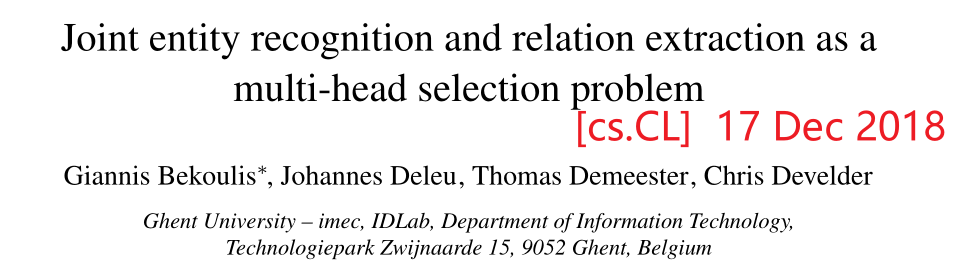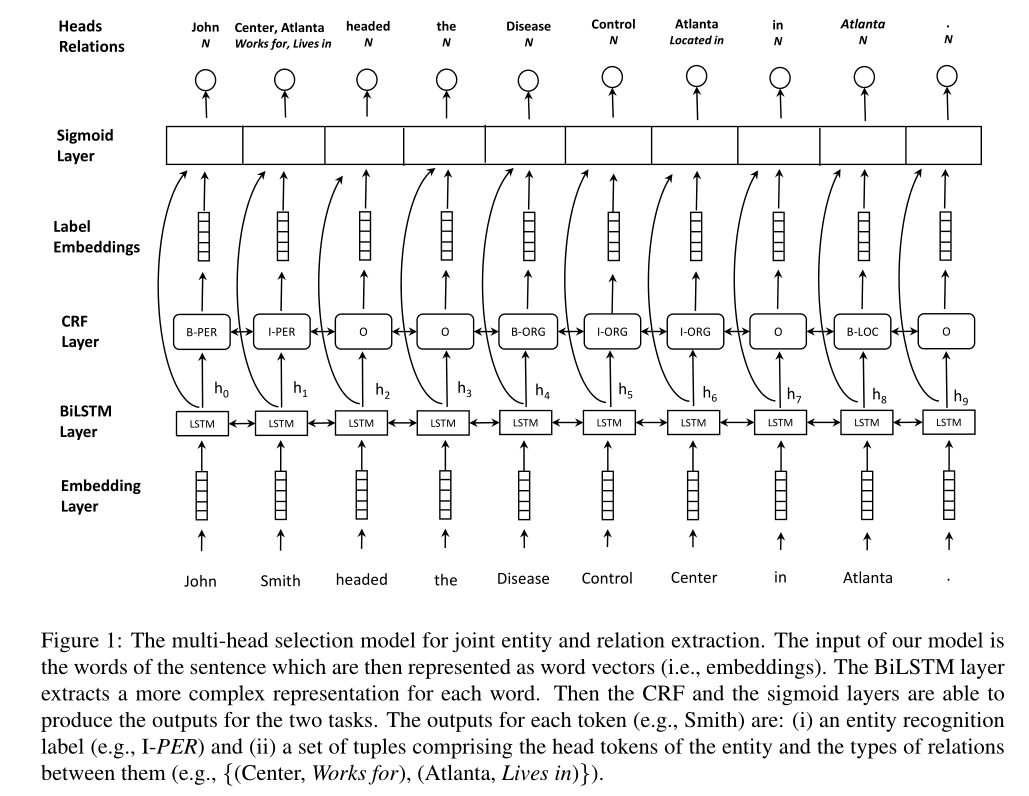# Joint entity recognition and relation extraction as a multi-head selection problemtfcode

# 1 Model1. 一个是实体识别标签（例如I-PER表示token的类型为PER）
2. 另一个是集合，这个集合包含三元组的头实体token和两个实体之间的关系(如：{(Center, Work for), (Atlanta, Lives in)})
3. note: 本文采用基于token的编码，所以让一个实体的最后一个单词作为实体的头部
1. 例如：“John Smith”和“Disease Control Center”之间有一种"works for"关系，本文没有链接所有实体的token，而是链接Smith，Works for，Center
4. 对于没有关系的情况，本文引入N标签

• 输入：一个token序列
• 词嵌入+字符嵌入

## 1.2 BiLSTM encoding layer

h i = [ h → i ; h ← i ] h_i=[\overset{\rightarrow}{h}_i;\overset{\leftarrow}{h}_i]

## 1.3 NER

s e ( h i ) = V e f ( U e h i + b e ) s^e(h_i) = V^ef(U^eh_i+b^e)

P r ( t a g ∣ w i ) = s o f t m a x ( s ( h i ) ) P r ( t a g ∣ w i ) ∈ R p Pr(tag|w_i)=softmax(s(h_i))\\ Pr(tag|w_i)\in R^p

z i = [ h i ; g i ] z_i=[h_i;g_i]

## 1.4 Relation extraction as multi-head selection

s r ( z j , z i , r k ) = V r f ( U r z j + W r z i + b r ) s^r(z_j,z_i,r_k)=V^rf(U^rz_j+W^rz_i+b^r)

P r ( h e a d = w j , l a b e l = r k ∣ w i ) = σ ( s r ( z j , z i , r k ) ) (1) Pr(head=w_j,label=r_k|w_i)=\sigma(s^r(z_j,z_i,r_k)) \tag{1}

L r e l = ∑ i = 0 n ∑ j = 0 m − l o g P r ( h e a d = y i , j , r e l a t i o n = r i , j ∣ w i ) \mathcal{L}_{rel}=\sum_{i=0}^{n}\sum_{j=0}^{m}-logPr(head=y_{i,j},relation=r_{i,j}|w_i)

L N E R + L r e l \mathcal{L}_{NER}+ \mathcal{L}_{rel}

# 2 启示

1. 从论文中没有读懂到底怎么抽取尾实体的
2. 也可以选择先抽头实体，再抽尾实体和关系，也是多头选择
3. 论文太长了，34页

10-02307
04-083303
07-122843
01-265196
03-043045
07-041万+
07-03879
11-191891
10-22938
02-0781
12-153870
10-304050
03-06392
01-27772
02-172503
07-03249

### “相关推荐”对你有帮助么？

•非常没帮助
•没帮助
•一般
•有帮助
•非常有帮助被折叠的  条评论 为什么被折叠?到【灌水乐园】发言¥2 ¥4 ¥6 ¥10 ¥20余额支付 (余额：-- )扫码支付获取中扫码支付点击重新获取扫码支付1.余额是钱包充值的虚拟货币，按照1:1的比例进行支付金额的抵扣。
2.余额无法直接购买下载，可以购买VIP、C币套餐、付费专栏及课程。余额充值